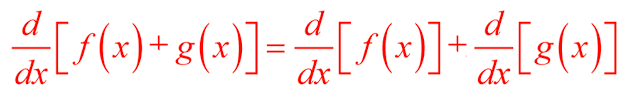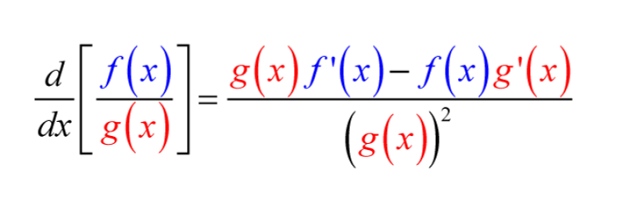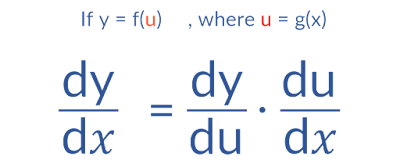# How to find dy/dx of a function

Derivatives in mathematics are a type of calculus which is used to find the rate at which a quantity changes with respect to another quantity. It is used to measure the sensitivity of a function to changes in its inputs. Derivatives are used to calculate the slope of a function at any given point, and to calculate the maximum or minimum value of a function.

The process of obtaining the derivatives of a function is called the differentiation. There are many ways of finding dy/dx of any function. As we move on higher class, we will keep on discovery many new methods of finding dy/dx or let's say derivative of a function.

Today we are going to have a look at the most commonly used techniques to find dy/dx of a function. We will discuss each topic briefly with an example so keep reading this till the very end so that you won't miss out any techniques.

## How to find dy/dx of a functionThe Derivatives chapter falls under Calculus sections. In derivatives chapter we will learn about many methods of finding derivative like ways of finding derivative of logarithmic functions, derivatives of trigonometric functions, derivatives of exponential functions and many more.

To find derivatives of any kind of function we mostly use 4 simple rules or let's say techniques. We can easily find dy/dx of a function using the following 4 rules of differentiation.

### 1. First principle or by definition

There is a term known as "derivative by first principle" which refers to the use of algebra to find a general expression for the slope of a curve. It is also known as "delta method". A small change in the value of a variable is known as increment. The small increment in 'X' is represented by '∆X' and small increment in 'Y' is represented by '∆Y'. Let's find dy/dx of a function using the first principle.

Derivative of function using first principle
Q.  Xⁿ
Solution:
Let y = xⁿ .................... (i)
Let ∆x be a small increment in x and ∆y a corresponding increment in y. Then,
y+ ∆y = (x+∆x)ⁿ ................. (ii)
Subtracting (i) from (ii), we get
or, ∆y =  (x+∆x)ⁿ - xⁿ
or, ∆y/∆x = {(x+∆x)ⁿ - xⁿ }/∆x
Now,
dy/dx = ᴸᶦᵐ∆x →0 ∆y/∆x
= ᴸᶦᵐ∆x →0 {(x+∆x)ⁿ - xⁿ }/{(x+∆x) - x}
= nxⁿ⁻¹  [∴ ᴸᶦᵐ∆x →a {(xⁿ - aⁿ)/x - a} = nxⁿ⁻¹]

### 2. The Power Rule

The power rule states that the derivative of a function of the form f(x) = x^n, where n is a real number, is equal to nx^{n-1}. It is used to calculate the slope of polynomial functions and any other function that contains an exponent with a real number. In other words, it helps to take the derivative of a variable raised to a power (exponent).The Power Rule Formula

Q. Find the derivative of x²
Solution:### 3. The Sum Rule

In terms of derivatives, the sum rule states that the derivative of a sum is equal to the sum of the derivatives.The Sum Rule Formula

Worked Example

Q. Find the derivative of 3x⁵ + 4x⁴
Solution:

### 4. The Difference Rule

According to the Difference Rule, the derivative of the difference between two functions is the derivative their difference.The Difference Rule Formula

Worked Example

Q. Find the derivative of f(x) = x³ - 5x²
Solution:

### 5. The Product Rule

The word 'product' makes us clear what this rule is about. The term 'product' means two functions are being multiplied together.

The product rule allows us to find the derivative of two differentiable functions which are being multiplied together using both our knowledge of the power rule and the sum and difference rule for derivatives in order to find the derivative of these two differentiable functions.

Basically, the rule is that the derivative of a product of two functions is a product of the first function multiplied by the derivative of the second function multiplied by the derivative of the first function.The Product Rule Formula

To better understand this formula, let's take a closer look at a few examples using color-coding to make it easier to understand.

Worked Example

Q. Find the derivative of (3 - x²).(x + 4)
Solution:

### 6. The Quotient Rule

Acccoding to the Quotient rule, it is the derivative of the function in the numerator times the function in the denominator minus the derivative of the function in the denominator times the function in the numerator, all divided by the square of the function in the denominator.The Quotient Rule Formula

Worked Example

Q. Find the derivative of (5x + 1) / (3x + 4)
Solution:

### 7. The Chain Rule

The chain rule is used to calculate the derivative of a composite function. The chain rule formula states that dy/dx = dy/du × du/dx. In words, differentiate the outer function while keeping the inner function the same then multiply this by the derivative of the inner function.The Chain Rule Formula

Worked Example

Q. Find the derivative of (4x³ - 5x + 6x - 7)¹⁰
Solution:

## Derivative of all Trigonometric Functions

While finding derivative, you may sometimes have find the differentiation of trigonometric functions. In such cases, you must know the derivatives of all trigonometric functions otherwise you won't be able to solve any of them.

Here are the derivatives of the common trigonometric functions:

S.NTrigonometric Functions Derivatives
1Sin(x)Cos(x)
2Cos(x)-Sin(x)
3Tan(x)Sec²(x)
4Cot(x)-Cosec²(x)
5Sec(x)-Sec(x).Tan(x)
6Cosec(x)-Cosec(x).Cot(x)
7Sin(ax)aCos(ax)
8Cos(ax)-aSin(ax)
9Tan(ax)aSec²(ax)
10Cot(ax)-aCosec²(ax)
11Sec(ax)-aSec(ax).Tan(ax)
12Cosec(ax)-aCosec(ax).Cot(ax)

## How to find the derivative of a polynomial function?

If you want to find the derivative of a polynomial function, you can use the power rule. The power rule states that the derivative of a term with a variable raised to a power is equal to the product of the power and the coefficient of the term, with the power reduced by 1.

For example, if you have the polynomial function f(x) = 4x² + 3x + 1, you can find its derivative by taking the derivative of each term separately. Applying the power rule, we get:

• The derivative of 4x² is 8x (power rule: 2 * 4x^(2-1) = 8x)
• The derivative of 2x is 2 (power rule: 1 * 3x^(1-1) = 1 * 3)
• The derivative of 1 is 0 (the derivative of a constant is always 0)
• Therefore, the derivative of f(x) is f'(x) = 6x + 2.

It's important to remember that the power rule only applies to terms with variables raised to a power. If your polynomial function includes other types of terms, such as trigonometric functions or logarithms then you need to use other rules to find their derivatives.

## How to find the derivative of a function with multiple variables?

Inorder to find the derivative of a function with multiple variables, you need to use partial derivatives. A partial derivative is the derivative of a function with respect to one of its variables, while holding all other variables constant.

Now to find the partial derivative of a function with respect to one variable, you have to differentiate the function with respect to that variable, treating all other variables as constants. This process is repeat for each variable in the function.

For example, if you have a function f(x,y) = x^2y + 3x - 2y, the partial derivative of f with respect to x would be 2xy + 3, and the partial derivative of f with respect to y would be x² - 2.

Once you have find all the partial derivatives, you can combine them into a vector known as the gradient vector. The gradient vector gives you the direction and magnitude of the maximum rate of change of the function at any given point.

## How do I find the derivative of a composite function?

If you want to find the derivative of a composite function then you can use the chain rule. We already have discussed about the chain rule before but also let's go in more depth.

The chain rule states that if y = f(g(x)), then dy/dx = dy/dg * dg/dx, where dy/dg is the derivative of the outer function f with respect to the inner function g, and dg/dx is the derivative of the inner function g with respect to x.

To apply the chain rule, you need to first identify the inner and outer functions of the composite function. Then, take the derivative of the outer function with respect to the inner function, and multiply it by the derivative of the inner function with respect to x.

For example, if you have a composite function y = f{g(x)} where f(u) = sin(u) and g(x) = 2x + 1, then you would first find the derivative of f with respect to g, which is cos{g(x)}. Then, you would find the derivative of g with respect to x, which is 2. Finally, you would multiply these two derivatives together to get dy/dx = cos{g(x)} * 2.

## Conclusion

No matter the complexity of the equation, the most important part of finding dy/dx is to remember the basic rules and apply them consistently. It can be helpful to break down problems into smaller parts, and to make sure to use the correct order of operations. With practice and dedication, anyone can learn how to find dy/dx.

Thank you for taking the time to read my blog article! I hope it has provided you with valuable insight and knowledge.

How to find dy/dx?

In general, to find dy/dx of a function, you need to take the derivative of the function y with respect to x using differentiation rules.

What is the difference between dy/dx and d/dx?

Both dy/dx and d/dx are related to differentiation, which is a fundamental concept in calculus. But, d/dx is used to find the derivative of a function with respect to its independent variable, while dy/dx is used to find the derivative of a dependent variable with respect to an independent variable.

Can I use the power rule to find the derivative of any function?

No, the power rule can be used to find the derivative of function which is in the form f(x) = x^n, where n is a constant. The power rule states that the derivative of f(x) = x^n is f'(x) = n*x^(n-1).

Can I find dy/dx using the product rule or quotient rule?

Yes, you can use both the product rule and the quotient rule to find dy/dx depending on the function you are dealing with. The product rule is used when you have two functions being multiplied together. On the other hand, the quotient rule is used when you have one function divided by another function.

No Comment